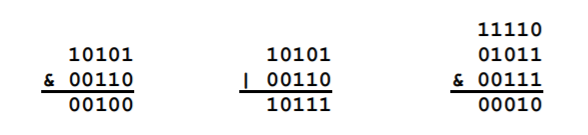BOI 2006 P1 - Bitwise Expressions

View as PDF

Points: 15 (partial)
Time limit: 10.0s
Memory limit: 512M

Problem type

In signal processing, one is sometimes interested in the maximum value of a certain expression, containing bitwise AND and OR operators, when the variables are integers in certain ranges. You are to write a program that takes such an expression and the range of each variable as input and determines the maximum value that the expression can take.

For simplicity, the expression is of a specific form, namely a number of subexpressions in parentheses separated by the bitwise AND operator (denoted). Each subexpression consists of one or more variables separated by the bitwise OR operator (denoted). Using this convention, it is possible to completely specify the expression by giving the number of subexpressions and, for each subexpression, the number of variables in the subexpression. The variables are simply numbered according to their occurrence in the expression.

An example will clarify this. If the number of subexpressions isand the number of variables in each subexpression isand, then the expression will beThe bitwise operators are defined in the common way. For example, to perform the operation, we first write down the binary form of the numbers (operands):and(sinceand). Every binary digit in the result now depends on the corresponding digit in the operands: if it isin both operands, the resulting digit will be one, otherwise it will be zero. As is illustrated below to the left, the resulting value is. If instead we want to calculate, the procedure is the same except that the resulting digit will be one if the corresponding digit is one in any of the operands, and thus it will be zero only in the case that the digit is zero in both operands. As is illustrated below in the center, the result is. The generalization to more than two operands is straightforward. The rightmost example below illustrates that.Input

In the first line, two integersandare given, whereis the total number of variablesandis the number of subexpressions. In the next line,integersare given, whereis the number of variables in the i-th subexpression. Theare all greater than or equal toand their sum equals. Each of the following N lines contains two integersand, specifying the range of the j-th variable in the expression according toOutput

The output should be one row with a single integer: the maximum value that the expression can take.

Example

Assume that we want to limit the values of the eight variables in the expression above according toand.

Sample Input

8 4
3 1 2 2
2 4
1 4
0 0
1 7
1 4
1 2
3 4
2 3

If writing in binary notation one of the best assignments gives the expressionfrom which we note that all subexpressions may become equal toexcept the third.

Sample Output

6

Inof the test cases, the number of possible assignments is less than one million.